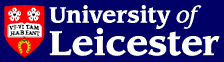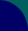# School of Mathematics & Computer Science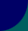# Technical Reports 2004

## Summary

2004/01 P. Houston, I. Perugia, A. Schneebeli and D. Schotzau.

Interior Penalty Method for the Indefinite Time-Harmonic Maxwell Equations

2004/02 P. Houston, D. Schotzau and T. Wihler.

Mixed hp-Discontinuous Galerkin Finite Element Methods for the Stokes Problem in Polygons

2004/03 R. Hartmann and P. Houston.

Adaptive Discontinuous Galerkin Finite Element Methods with Interior Penalty for the Compressible Navier-Stokes Equations

2004/04 P.Houston, I. Perugia, A. Schneebeli and D. Schotzau.

Discontinuous Galerkin Methods for the Time-Harmonic Maxwell Equations

2004/05 P. Houston, Ch. Schwab and E. Suli.

On the Design of hp-Adaptive Finite Element Methods for Elliptic Partial Differential Equations.

2004/06 Yifeng Chen and Zhiming Liu.

Integrating Temporal Logics

2004/07 Edward L. Green and Nicole Snashall.

The Hochschild Cohomology Ring Modulo Nilpotence of a Stacked Monomial Algebra

2004/08 Aslak Bakke Buan, Robert Marsh, Markus Reineke, Idun Reiten and Gordana Todorov.

Tilting Theory and Cluster Combinatorics

2004/09 Damian Brown, Leevan Ling, Edward Kansa and Jeremy Levesley.

On Approximate Cardinal Preconditioning Methods for Solving PDEs with Radial Basis Functions

2004/10 Aslak Bakke Buan, Robert Marsh and Idun Reiten.

Cluster-Tilted Algebras

2004/11 Rob Brownlee, Will Light, D. Schotzau and T.P. Wihler.

Approximation Orders for Interpolation by Surface Splines to Rough Functions

2004/12 Gabriel Davis.

Finiteness Conditions on the Ext-algebra of a Cycle Algebra

2004/13 Rob Brownlee and Jeremy Levesley.

A scale of improved error estimates for radial approximation in Euclidean space and on spheres

2004/14 J. Levesley and X. Sun.

Approximation in Native Spaces

2004/15 Rob Brownlee.

Error estimates for interpolation of rough data using the scattered shifts of a radial basis function

2004/16 Jurgen Muller.

Invariant Theory of Finite Groups

2004/17 P. Houston, D. Schotzau and T.P. Wihler.

hp-Adaptive Discontinuous Galerkin Finite Element Methods for the Stokes ProblemError estimates for interpolation of rough data using the scattered shifts of a radial basis function

2004/18 P. Houston, I. Perugia and D. Schotzau.

A Review of Discontinuous Galerkin Methods for Maxwell's Equation D. Schotzau T.P. Wihlers in Frequency-Domain

2004/19 P. Houston, I. Perugia, A. Schneebeli and D. Schotzau.

Mixed Discontinuous Galerkin Approximation of the Maxwell Operator: The Indefinite Case.

2004/20 P. Houston, I. Perugia and D. Schotzau.

Recent Developments in Discontinuous Galerkin Methods for the Time-Harmonic Maxwell's Equations.

2004/21 G.N. Milstein and M.V. Tretyakov.

Numerical algorithms for forward-backward stochastic differential equations connected with semilinear parabolic equations

2004/22 Duncan W. Parkes, V. Yu. Shavrukov and Richard M. Thomas.

Monoid Presentations of Groups by Finite Special String-Rewriting Systems

2004/23 Derek F. Holt, Sarah Rees, Claas E. Rover and Richard M. Thomas.

Groups with context-free co-word problem

2004/24 P. Houston, J. Robson and E. Suli.

Discontinuous Galerkin finite element approximation of quasilinear elliptic boundary value problems I: The scalar case.

2004/25 Edward L. Green, Nicole Snashall and Oeyvind Solberg.

The Hochschild cohomology ring modulo nilpotence of a monomial algebra

2004/26 R. A. Brownlee.

Error Estimates for Interpolation of Rough and Smooth Functions using Radial Basis Functions

2004/27 P. Kosiuczenko.

Proof Transformation via Interpretation Functions

2004/28 I. Ulidowski (editor) .

Preliminary Proceedings
6th AMAST Workshop on Real-Time Systems
ARTS 2004
Stirling, U.K., July 12, 2004

2004/29 Y. Chen.

A Language of Flexible Objects

2004/30 J. Hunton and Bjorn Schuster.

Subalgebras of Group Cohomology Defined by Infinite Loop Spaces

2004/31 G.N. Milstein, M.V. Tretyakov and M.Hoffmann.

Numerical integration of stochastic differential equations with nonglobally Lipschitz coefficients

2004/32 P. Houston, D. Schotzau and T.P. Wihler.

Energy Norm A Posteriori Error Estimation of hp-Adaptive Discontinous Galerkin Methods for Elliptic Problems

2004/33 Tim Hardcastle.

Normal and characteristic strucute in quasigroups and loops - PhD Thesis

2004/34 P. Houston, D. Schotzau and T.P. Wihler.

An hp-Adaptive Mixed Discontinuous Galerkin FEM for Nearly Incompressible Linear Elasticity

2004/35 G.N. Milstein and M.V. Tretyakov.

Solving forward-backward stochastic differential equations and related quasilinear parabolic equations

2004/36 N.Snashall, K Erdmann, E L Green and R Taillefer.

Representation Theory of the Drinfel'd Doubles of a Family of Hopf Algebras

2004/37 Michael Hoffmann and Richard M.Thomas.

A geometric characterization of automatic semigroups

2004/38 Graham P.Oliver and Richard M.Thomas.

Finitely generated groups with automatic presentations

2004/39 Stephen R. Lakin and Richard M.Thomas.

Context-sensitive decision problems in groups

2004/40 Aslak Bakke Buan, Robert Marsh and Idun Reiten.

Cluster mutation via quiver representations

## Full details

2004/01 P. Houston, I. Perugia, A. Schneebeli and D. Schotzau.

Interior Penalty Method for the Indefinite Time-Harmonic Maxwell Equations

In this paper, we introduce and analyze the interior penalty discontinuous Galerkin method for the numerical discretization of the {\em indefinite} time-harmonic Maxwell equations in high-frequency regime. Based on suitable duality arguments, we derive a-priori error bounds in the energy norm and the $L^2$-norm. In particular, the error in the energy norm is shown to converge with the optimal order ${\mathcal O}(h^{\min\{s,\ell\}})$ with respect to the mesh size~$h$, the polynomial degree~$\ell$, and the regularity exponent~$s$ of the analytical solution. Under additional regularity assumptions, the $L^2$-error is shown to converge with the optimal order ${\mathcal O}(h^{\ell+1})$. The theoretical results are confirmed in a series of numerical experiments.

Available as: gzipped PostScript (.ps.gz)

2004/02 P. Houston, D. Schotzau and T. Wihler.

Mixed hp-Discontinuous Galerkin Finite Element Methods for the Stokes Problem in Polygons

No abstract.

Available as: gzipped PostScript (.ps.gz)

2004/03 R. Hartmann and P. Houston.

Adaptive Discontinuous Galerkin Finite Element Methods with Interior Penalty for the Compressible Navier-Stokes Equations

No abstract.

Available as: gzipped PostScript (.ps.gz)

2004/04 P.Houston, I. Perugia, A. Schneebeli and D. Schotzau.

Discontinuous Galerkin Methods for the Time-Harmonic Maxwell Equations

No abstract.

Available as: gzipped PostScript (.ps.gz)

2004/05 P. Houston, Ch. Schwab and E. Suli.

On the Design of hp-Adaptive Finite Element Methods for Elliptic Partial Differential Equations.

We introduce an $hp$--adaptive finite element algorithm based on a combination of reliable and efficient residual error indicators and a new $hp$--extension control technique which assesses the local regularity of the underlying analytical solution on the basis of its local Legendre series expansion. Numerical experiments confirm the robustness and reliability of the proposed algorithm.

Available as: gzipped PostScript (.ps.gz)

2004/06 Yifeng Chen and Zhiming Liu.

Integrating Temporal Logics

In this paper, we study the predicative semantics of different temporal logics and the relationships between them. We use a notation called generic composition to simplify the manipulation of predicates. The modalities of possibility and necessity become generic composition and its inverse of converse respectively. The relationships between different temporal logics are also characterised as such modalities. Formal reasoning is carried out at the level of predicative semantics and supported by the higher-level laws of generic composition and its inverse. Various temporal domains are unified under a notion called resource cumulation. Temporal logics based on these temporal domains can be readily defined, and their axioms identified. The formalism provides a framework in which human experience about system development can be formalised as refinement laws. The approach is demonstrated in the transformation from Duration Calculus to Temporal Logic of Actions. A number of common design patterns are studied. The refinement laws identified are then applied to the case study of water pump controlling.

2004/07 Edward L. Green and Nicole Snashall.

The Hochschild Cohomology Ring Modulo Nilpotence of a Stacked Monomial Algebra

This paper studies the Hochschild cohomology of finite-dimensional monomial algebras. If A = KQ/I with I an admissible monomial ideal, then we give sufficient conditions for the existence of an embedding of K[x_1, ..., x_r]/( x_ax_b for a \neq b) into the Hochschild cohomology ring HH^*(A). We also introduce stacked algebras, a new class of monomial algebras which includes Koszul and D-Koszul monomial algebras. If A is a stacked algebra, we prove that HH^*(A)/N is isomorphic to K[x_1, ..., x_r]/( x_ax_b for a \neq b), where N is the ideal in HH^*(A) generated by the homogeneous nilpotent elements. In particular, this shows that the Hochschild cohomology ring of A modulo nilpotence is finitely generated as an algebra.

2004/08 Aslak Bakke Buan, Robert Marsh, Markus Reineke, Idun Reiten and Gordana Todorov.

Tilting Theory and Cluster Combinatorics

We introduce a new category C, which we call the cluster category, obtained as a quotient of the bounded derived category D of the module category of a finite-dimensional hereditary algebra H over a field. We show that, in the simply-laced Dynkin case, C can be regarded as a natural model for the combinatorics of the corresponding Fomin-Zelevinsky cluster algebra. In this model, the tilting modules correspond to the clusters of Fomin-Zelevinsky. Using approximation theory, we investigate the tilting theory of C, showing that it is more regular than that of the module category itself, and demonstrating an interesting link with the classification of self-injective algebras of finite representation type. This investigation also enables us to conjecture a generalisation of APR-tilting.

Available as: gzipped PostScript (.ps.gz)

2004/09 Damian Brown, Leevan Ling, Edward Kansa and Jeremy Levesley.

On Approximate Cardinal Preconditioning Methods for Solving PDEs with Radial Basis Functions

The approximate cardinal basis function (ACBF) preconditioning technique has been used to solve partial differential equations (PDEs) with radial basis functions (RBFs). In \cite{LK02}, a preconditioning scheme that is based upon constructing the least-squares approximate cardinal basis function from linear combinations of the RBF-PDE matrix elements has shown very attractive numerical results. This preconditioning technique is sufficiently general that it can be easily applied to many differential operators.

In this paper, we review the ACBF preconditioning techniques previously used for interpolation problems and investigate a class of preconditioners based on the one proposed in \cite{LK02} when a cardinality condition is enforced on different subsets. We numerically compare the ACBF preconditioners on several numerical examples of Poisson's, modified Helmholtz and Helmholtz equations, as well as a diffusion equation and discuss their performance.

2004/10 Aslak Bakke Buan, Robert Marsh and Idun Reiten.

Cluster-Tilted Algebras

We introduce a new category C, which we call the cluster category, obtained as a quotient of the bounded derived category D of the module category of a finite-dimensional hereditary algebra H over a field. We show that, in the simply-laced Dynkin case, C can be regarded as a natural model for the combinatorics of the corresponding Fomin-Zelevinsky cluster algebra. In this model, the tilting modules correspond to the clusters of Fomin-Zelevinsky. Using approximation theory, we investigate the tilting theory of C, showing that it is more regular than that of the module category itself, and demonstrating an interesting link with the classification of self-injective algebras of finite representation type. This investigation also enables us to conjecture a generalisation of APR-tilting.

Available as: gzipped PostScript (.ps.gz)

2004/11 Rob Brownlee, Will Light, D. Schotzau and T.P. Wihler.

Approximation Orders for Interpolation by Surface Splines to Rough Functions

In this paper we consider the approximation of functions by radial basic function interpolants. There is a plethora of results about the asymptotic behaviour of the error between appropriately smooth functions and their interpolants, as the interpolation points fill out a bounded domain in R^d. In all of these cases, the analysis takes place in a natural function space dictated by the choice of radial basis function - the native space. In many cases, the native space contains functions possessing a certain amount of smoothness. We address the question of what can be said about these error estimates when the function being interpolated fails to have the required smoothness. These are the rough functions of the title. We limit our discussion to surface splines, as an exemplar of a wider class of radial basic functions, because we feel our techniques are most easily seen and understood in this setting.

Available as: gzipped PostScript (.ps.gz)

2004/12 Gabriel Davis.

Finiteness Conditions on the Ext-algebra of a Cycle Algebra

Let $A$ be a finite-dimensional algebra given by quiver and monomial relations. In [E.L. Green, D. Zacharia, Manuscripta Math.\ 85 (1994) 11-23] we see that the Ext-algebra of $A$ is finitely generated if and only if all the Ext-algebras of certain cycle algebras overlying $A$ are finitely generated. Here a cycle algebra $\Lambda$ is a finite-dimensional algebra given by quiver and monomial relations where the quiver is an oriented cycle. The main result of this paper gives necessary and sufficient conditions for the Ext-algebra of such a $\Lambda$ to be finitely generated; this is achieved by defining a computable invariant of $\Lambda$, the smo-tube. We also give necessary and sufficient conditions for the Ext-algebra of $\Lambda$ to be Noetherian.

2004/13 Rob Brownlee and Jeremy Levesley.

A scale of improved error estimates for radial approximation in Euclidean space and on spheres

We adapt Schaback's error doubling trick~\cite{schaback} to give error estimates for radial interpolation of functions with smoothness lying (in some sense) between that of the usual native space and the subspace with double the smoothness. We do this for both bounded subsets of $\R^d$ and spheres. As a step on the way to our ultimate goal we also show convergence of derivatives of the interpolation error

2004/14 J. Levesley and X. Sun.

Approximation in Native Spaces

Within the conventional framework of a native space structure, a smooth kernel generates a small native space, and radial basis functions" stemming from the smooth kernel are intended to approximate only functions from this small native space. Therefore their approximation power is quite limited. Recently, Narcowich, Schaback and Ward [NSW], and Narcowich and Ward [NW], respectively, have studied two approaches that have led to the empowerment of smooth radial basis functions in a larger native space. In the approach of [NW], the radial basis function interpolates the target function at some scattered (prescribed) points. In both approaches, approximation power of the smooth radial basis functions is achieved by utilizing spherical polynomials of a (possibly) large degree to form an intermediate approximation between the radial basis approximation and the target function. In this paper, we take a new approach. We embed the smooth radial basis functions in a larger native space generated by a less smooth kernel, and use them to approximate functions from the larger native space. Among other results, we characterize the best approximant with respect to the metric of the larger native space to be the radial basis function that interpolates the target function on a set of finite scattered points after the action of a certain multiplier operator. We also Michael Hoffmann Richard M.Thomas

In the study of automatic groups, the geometrical characterization of automaticity (in terms of the "fellow traveller property") plays a fundamental role. When we move to the study of automatic semigroups, we no longer have this simple formulation. The purpose of this paper is to give a general geometric characterization of automaticity in semigroups.

establish the error bounds between the best approximant and the target function.

2004/15 Rob Brownlee.

Error estimates for interpolation of rough data using the scattered shifts of a radial basis function

D. Schotzau T.P. Wihler

The error between appropriately smooth functions and their radial basis function interpolants, as the interpolation points fill out a bounded domain in $\R^d$, is a well studied artifact. In all of these cases, the analysis takes place in a natural function space dictated by the choice of radial basis function---the native space. The native space contains functions possessing a certain amount of smoothness. We address the question of what happens if the function being approximated is conspicuously rough.

2004/16 Jurgen Muller.

Invariant Theory of Finite Groups

This introductory lecture will be concerned with polynomial invariants of finite groups which come from a linear group action. We will introduce the basic notions of invariant theory, discuss the structural properties of invariant rings, comment on computational aspects, and finally present two applications from function theory and number theory.

Keywords are: polynomial rings, graded commutative algebras, Hilbert series, Noether normalization, Cohen-Macaulay property, primary and secondary invariants symmetric groups, reflection groups.

2004/17 P. Houston, D. Schotzau and T.P. Wihler.

hp-Adaptive Discontinuous Galerkin Finite Element Methods for the Stokes ProblemError estimates for interpolation of rough data using the scattered shifts of a radial basis function

In this paper, we derive an $hp$--version a posteriori error estimator for mixed discontinuous Galerkin finite element methods for the Stokes problem. The estimator is obtained by extending the $h$--version a posteriori analysis of  to the context of the $hp$--version of the finite element method. We then present a series of numerical experiments where we test the performance of the proposed error estimator within an automatic $hp$--adaptive refinement procedure.

Available as: gzipped PostScript (.ps.gz)

2004/18 P. Houston, I. Perugia and D. Schotzau.

A Review of Discontinuous Galerkin Methods for Maxwell's Equation D. Schotzau T.P. Wihlers in Frequency-Domain

In this paper, we review recent work on discontinuous Galerkin (DG) methods for the discretization of the time-harmonic Maxwell equations in frequency-domain, based on the interior penalty discretization of the curl-curl operator. Direct and mixed methods will be presented for low-frequency and high-frequency cases. The performance of the mixed DG method for the indefinite Maxwell problem in the high-frequency case is tested in a new set of numerical experiments carried out on a model problem with a singular solution.

Available as: gzipped PostScript (.ps.gz)

2004/19 P. Houston, I. Perugia, A. Schneebeli and D. Schotzau.

Mixed Discontinuous Galerkin Approximation of the Maxwell Operator: The Indefinite Case.

We present and analyze an interior penalty D. Schotzau T.P. Wihler method for the numerical discretization of the indefinite time-harmonic Maxwell equations in mixed form. The method is based on the mixed discretization of the curl-curl operator developed in  and can be understood as a non-stabilized variant of the approach proposed in . We show the well-posedness of this approach and derive optimal a-priori error estimates in the energy-norm as well as the $L^2$-norm. The theoretical results are confirmed in a series of numerical experiments.

Available as: gzipped PostScript (.ps.gz)

2004/20 P. Houston, I. Perugia and D. Schotzau.

Recent Developments in Discontinuous Galerkin Methods for the Time-Harmonic Maxwell's Equations.

In this article, we review recent work on discontinuous Galerkin (DG, for short) methods for the discretization of the time-harmonic Maxwell's equations, based on the interior penalty discretization of the curl-curl operator. Direct and mixed methods will be presented for both the low- and high-frequency cases. The performance of the proposed DG methods will be highlighted in a series of numerical examples with known analytical solutions.

Available as: gzipped PostScript (.ps.gz)

2004/21 G.N. Milstein and M.V. Tretyakov.

Numerical algorithms for forward-backward stochastic differential equations connected with semilinear parabolic equations

Efficient numerical algorithms are proposed for a class of forward-backward stochastic differential equations (FBSDEs) connected with semilinear parabolic partial differential equations. As in [J. Douglas, Jr., J. Ma, P. Protter, Ann. Appl. Prob., 6 (1996), 940--968.], the algorithms are based on the known four-step scheme for solving FBSDEs. The corresponding semilinear parabolic equation is solved by layer methods which are constructed by means of a probabilistic approach. The derivatives of the solution u of the semilinear equation are found by finite differences. The forward equation is simulated by mean-square methods of order 1/2 and 1. Corresponding convergence theorems are proved. Along with the algorithms for FBSDEs on a fixed finite time interval, we also construct algorithms for FBSDEs with random terminal time. The results obtained are supported by numerical experiments.

AMS 2000 subject classification. Primary 60H35; secondary 65C30, 60H10, 62P05.

Keywords. Forward-backward stochastic differential equations, numerical integration, mean-square convergence, semilinear partial differential equations of parabolic type.

2004/22 Duncan W. Parkes, V. Yu. Shavrukov and Richard M. Thomas.

Monoid Presentations of Groups by Finite Special String-Rewriting Systems

We show that the class of groups which have monoid presentations by means of finite special $\lambda$-confluent string-rewriting systems strictly contains the class of plain groups (the groups which are free products of a finitely generated free group and finitely many finite groups), and that any group which has an infinite cyclic central subgroup can be presented by such a string-rewriting system if and only if it is the direct product of an infinite cyclic group and a finite cyclic group.

2004/23 Derek F. Holt, Sarah Rees, Claas E. Rover and Richard M. Thomas.

Groups with context-free co-word problem

We study the class of co-context-free groups. We define a co-context-free group to be one whose co-word problem (the complement of its word problem) is context-free. This class is larger than the subclass of context-free groups, being closed under the taking of finite direct products, restricted standard wreath products with context-free top groups, and passing to finitely generated subgroups and finite index overgroups. But we do not know of other examples of co-context-free groups. We prove that the only examples amongst polycyclic groups or the Baumslag-Solitar groups are virtually abelian. We do this by proving that languages with certain purely arithmetical properties cannot be context-free; this result may be of independent interest.

2004/24 P. Houston, J. Robson and E. Suli.

Discontinuous Galerkin finite element approximation of quasilinear elliptic boundary value problems I: The scalar case.

We develop a one--parameter family of $hp$--version discontinuous Galerkin finite element methods, parameterised by $\theta \in [-1,1]$, for the numerical solution of quasilinear elliptic equations in divergence--form on a bounded open set $\Omega \subset \mathbb{R}^d$, $d \geq 2$. In particular, we consider the analysis of the family for the equation $-\nabla\cdot\left\{\mu(x,|\nabla u|)\nabla u\right\} = f(x)$ subject to mixed Dirichlet--Neumann boundary conditions on $\partial \Omega$. It is assumed that $\mu$ is a real--valued function, $\mu \in C(\bar{\Omega}\times[0,\infty))$, and there exist positive constants $m_\mu$ and $M_\mu$ such that $m_{\mu} (t-s) \leq \mu(x,t)t - \mu(x,s)s \leq M_{\mu} (t-s)$ for $t \geq s \geq 0$ and all $x \in \bar{\Omega}$. Using Brouwer's Fixed Point Theorem, for any value of $\theta \in [-1,1]$ the corresponding method is shown to have a unique solution $u_{\rm DG}$ in the finite element space. If $u \in C^1(\Omega) \cap H^k(\Omega)$, $k \geq 2$, then, with discontinuous piecewise polynomials of degree $p\geq 1$, the error between $u$ and $u_{\rm DG}$, measured in the broken $H^1(\Omega)$--norm, is $\mathcal{O}(h^{s-1}/p^{k-3/2})$, where $1 \leq s \leq \min\{p+1,k\}$.

Available as: gzipped PostScript (.ps.gz)

2004/25 Edward L. Green, Nicole Snashall and Oeyvind Solberg.

The Hochschild cohomology ring modulo nilpotence of a monomial algebra

For a finite dimensional monomial algebra A over a field K we show that the Hochschild cohomology ring of A modulo the ideal generated by homogeneous nilpotent elements is a commutative finitely generated K-algebra of Krull dimension at most one. This finite generation was conjectured to be true for any finite dimensional algebra over a field by N. Snashall and O. Solberg in "Support varieties and Hochschild cohomology rings", Proc. London Math. Soc. 88 (2004) 705-732.

2004/26 R. A. Brownlee.

Error Estimates for Interpolation of Rough and Smooth Functions using Radial Basis Functions

In this thesis we are concerned with the approximation of functions by radial basis function interpolants. There is a plethora of results about the asymptotic behaviour of the error between appropriately smooth functions and their interpolants, as the interpolation points fill out a bounded domain in Euclidean space. In all of these cases, the analysis takes place in a natural function space dictated by the choice of radial basis function{\Mdash}the native space.

This work establishes $L_p$-error estimates, for $1\leq p \leq \infty$, when the function being interpolated fails to have the required smoothness to lie in the corresponding native space; therefore, providing error estimates for a class of rougher functions than previously known. Such estimates have application in the numerical analysis of solving partial differential equations using radial basis function collocation methods. At first our discussion focusses on the popular polyharmonic splines. A more general class of radial basis functions is admitted into exposition later on, this class being characterised by the algebraic decay of the Fourier transform of the radial basis function. The new estimates presented here offer some improvement on recent contributions from other authors by having wider applicability and a more satisfactory form. The method of proof employed is not restricted to interpolation alone. Rather, the technique provides error estimates for the approximation of rough functions for a variety of related approximation schemes as well.

For the previously mentioned class of radial basis functions, this work also gives error estimates when the function being interpolated has some additional smoothness. We find that the usual $L_p$-error estimate, for $1\leq p \leq \infty$, where the approximand belongs to the corresponding native space, can be doubled. Furthermore, error estimates are established for functions with smoothness intermediate to that of the native space and the subspace of the native space where double the error is observed.

2004/27 P. Kosiuczenko.

Proof Transformation via Interpretation Functions

Abstract: In this paper we prove several useful facts concerning term mappings, proof transformation and preservation of different logical systems by term mappings. This research is motivated by a practical approach to an automatic transformation of class invariants and pre- and post-conditions. We show a method of extending mappings from a set of terms to, what we call interpretation functions, which are defined on larger sets of terms obtained by substitution. We provide a sufficient condition for compositional functions to preserve proofs in equational logic, as well as proofs in other logical systems like propositional logic with modus ponens or proofs using resolution rule. Consequently, constraint transformation via interpretation functions preserves entailment relations in several logical systems.

2004/28 I. Ulidowski (editor) .

Preliminary Proceedings
6th AMAST Workshop on Real-Time Systems
ARTS 2004
Stirling, U.K., July 12, 2004

No abstract.

2004/29 Y. Chen.

A Language of Flexible Objects

In this paper, we introduce a new language called \flexibo. \flexibo\ is an executable object-oriented specification language designed for open-source software development with different levels of {\em trust} in a {\em decentralized} programming environment. \flexibo\ provides a number of new programming mechanisms for both systematic static analysis and dynamic control of {\em correctness}, {\em ownership} and {\em resources}. All language ingredients are values. Many programming constructs have their corresponding {\em mirror classes}, each of which can be extended to programmer-defined sub-mirrorclasses. Various language operators such as method invocation are overridable. Unlike other OO languages that follow specific binding rules, \flexibo\ allows programmers to choose the binding mechanism of a variable by explicitly denoting its binding direction. Variables are untyped in \flexibo. Types are introduced as constraints and checked in runtime. However, if \flexibo\ is used for language translation, the checkings are actually done in the compilation phase of the whole process. Programmers are allowed to define their own types. Each type system then corresponds to a particular \flexibo\ program. That means \flexibo\ programs are able to compile themselves: a source \flexibo\ program can be evaluated for type checking and translated to another programming language, if the type checking succeeds. Unlike other languages providing one particular set of language mechanisms, \flexibo\ is designed to represent various language mechanisms systematically and flexibly.

2004/30 J. Hunton and Bjorn Schuster.

Subalgebras of Group Cohomology Defined by Infinite Loop Spaces

We study natural subalgebras $Ch_E(BG;R)$ of group cohomology $H^*(BG;R)$ defined in terms of the infinite loop spaces in spectra $E$ and give representation theoretic descriptions of those based on $QS^0$ and the Johnson-Wilson theories $E(n)$. We describe the subalgebras arising from the Brown-Peterson spectra $BP$ and as a result give a simple reproof of Yagita's theorem that the image of $BP^*(BG)$ in $H^*(BG;F_p)$ is $F$-isomorphic to the whole cohomology ring; the same result is shown to hold with $BP$ replaced by any complex oriented theory $E$ with a non-trivial map of ring spectra $E\rightarrow HF_p$. We also extend our constructions to define subalgebras of $H^*(X;R)$ for any space $X$; when $X$ is a finite CW complex we show that the subalgebras $Ch_{E(n)}(X;R)$ give a natural unstable chromatic filtration of $H^*(X;R)$.

2004/31 G.N. Milstein, M.V. Tretyakov and M.Hoffmann.

Numerical integration of stochastic differential equations with nonglobally Lipschitz coefficients

We propose a new conception which allows us to apply any numerical method of weak approximation to stochastic differential equations (SDEs) with nonglobally Lipschitz coefficients. Following this conception, we do not take into account the approximate trajectories which leave a sufficiently large sphere. We prove that accuracy of any method of weak order $p$ is estimated by $\varepsilon +O(h^{p})$, where $\varepsilon$ can be made arbitrarily small with increasing the radius of the sphere. The results obtained are supported by numerical experiments.

AMS 2000 subject classification. Primary 60H35; secondary 65C30, 60H10.

Keywords. SDEs with nonglobally Lipschitz coefficients, numerical integration of SDEs in the weak sense.

2004/32 P. Houston, D. Schotzau and T.P. Wihler.

Energy Norm A Posteriori Error Estimation of hp-Adaptive Discontinous Galerkin Methods for Elliptic Problems

In this paper, we develop the a posteriori error estimation of hp-version interior penalty discontinuous Galerkin discretizations of elliptic boundary-value problems. Computable upper and lower bounds on the error measured in terms of a natural (mesh-dependent) energy norm are derived. The bounds are explicit in the local mesh sizes and approximation orders. A series of numerical experiments illustrate the performance of the proposed estimators within an automatic hp-adaptive refinement procedure.

Available as: gzipped PostScript (.ps.gz)

2004/33 Tim Hardcastle.

Normal and characteristic strucute in quasigroups and loops - PhD Thesis

In this thesis I shall be exploring the normal and characteristic structure of quasigroups and loops. In recent years there has been a revival of interest in the theory of loops and in particular in the relationship between the properties of a loop and the properties of its multiplication group; and several powerful new theorems have emerged which allow the structural properties of a loop to be related to its multiplication group. I shall combine these ideas with tools developed at the beginning of loop theory to produce some interesting new theorems, principally relating the order of a finite multiplication group to the structure of its loop.

2004/34 P. Houston, D. Schotzau and T.P. Wihler.

An hp-Adaptive Mixed Discontinuous Galerkin FEM for Nearly Incompressible Linear Elasticity

We develop the a posteriori error estimation of mixed hp-version discontinuous Galerkin finite element methods for nearly incompressible elasticity problems. Computable upper and lower bounds on the error measured in terms of a natural (mesh-dependent) energy norm are derived. The bounds are explicit in the local mesh sizes and approximation orders, and are independent of the locking parameter. A series of numerical experiments are presented which demonstrate the performance of the proposed error estimator within an automatic hp-adaptive refinement procedure.

2004/35 G.N. Milstein and M.V. Tretyakov.

Solving forward-backward stochastic differential equations and related quasilinear parabolic equations

Efficient numerical algorithms for a class of forward-backward stochastic differential equations (FBSDEs) and related quasilinear parabolic partial differential equations (quasilinear PDEs) are proposed. The corresponding quasilinear parabolic equation is solved by new layer methods which are constructed by means of a probabilistic approach. Efficiency of the methods is achieved due to replacing derivatives of the solution to the quasilinear equation by finite differences. The proposed algorithms for solving FBSDEs are based on the four-step scheme of Ma, Protter, Yong. Convergence theorems are proved. Results of some numerical experiments are presented.

AMS 2000 subject classification. Primary 60H35; secondary 65C30, 60H10, 35K55.

Keywords. Forward-backward stochastic differential equations, numerical integration, mean-square convergence, quasilinear partial differential equations of parabolic type.

2004/36 N.Snashall, K Erdmann, E L Green and R Taillefer.

Representation Theory of the Drinfel'd Doubles of a Family of Hopf Algebras

We investigate the Drinfeld doubles of a certain family of Hopf algebras. We determine their simple modules and their indecomposable projective modules, and we obtain a presentation by quiver and relations of these Drinfeld doubles, from which we deduce properties of their representations, including their Auslander-Reiten quivers. We then determine decompositions of their tensor products of most of the representations described, and in particular give a complete description of the tensor product of two simple modules. This study also leads to explicit examples of Hopf bimodules over the original Hopf algebras.

2004/37 Michael Hoffmann and Richard M.Thomas.

A geometric characterization of automatic semigroups

In the study of automatic groups, the geometrical characterization of automaticity (in terms of the "fellow traveller property") plays a fundamental role. When we move to the study of automatic semigroups, we no longer have this simple formulation. The purpose of this paper is to give a general geometric characterization of automaticity in semigroups.

2004/38 Graham P.Oliver and Richard M.Thomas.

Finitely generated groups with automatic presentations

A structure is said to be computable if its domain can be represented by a set which is accepted by a Turing machine and if its relations can then be checked using Turing machines. Restricting the Turing machines in this defintion to finite automata gives us a class of structures with a particularly simple computational structure; these structures are said to have automatic presentations. Given their nice algorithmic properties, these have been of interest in a wide variety of areas. An area of particular interest has been the classification of automatic structures. One of the prime examples has been the class of groups. We give a complete characterization in the case of finitely generated groups and show that such a group has an automatic presentation if and only if it is virtually abelian.

2004/39 Stephen R. Lakin and Richard M.Thomas.

Context-sensitive decision problems in groups

There already exists classifications of those groups which have regular, context-free or recursively enumerable word problem. The only remaining step in the Chomsky heirarchy is to consider those groups with a context-sensitive word problem. In this paper we consider this problem and prove some results about these groups. We also establish some results about other context-sensitive decision problems in groups.

2004/40 Aslak Bakke Buan, Robert Marsh and Idun Reiten.

Cluster mutation via quiver representations

Matrix mutation appears in the definition of cluster algebras of Fomin and Zelevinsky. We give a representation theoretic interpretation of matrix mutation, using tilting theory in cluster categories of hereditary algebras. Using this, we obtain a representation theoretical interpretation of cluster mutation in case of acyclic cluster algebras of finite type.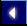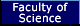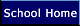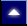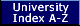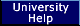Author: Steve Lakin (srl10@mcs.le.ac.uk).
Author: H. Thomas (H.Thomas@mcs.le.ac.uk).